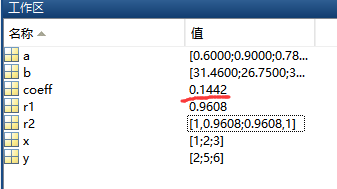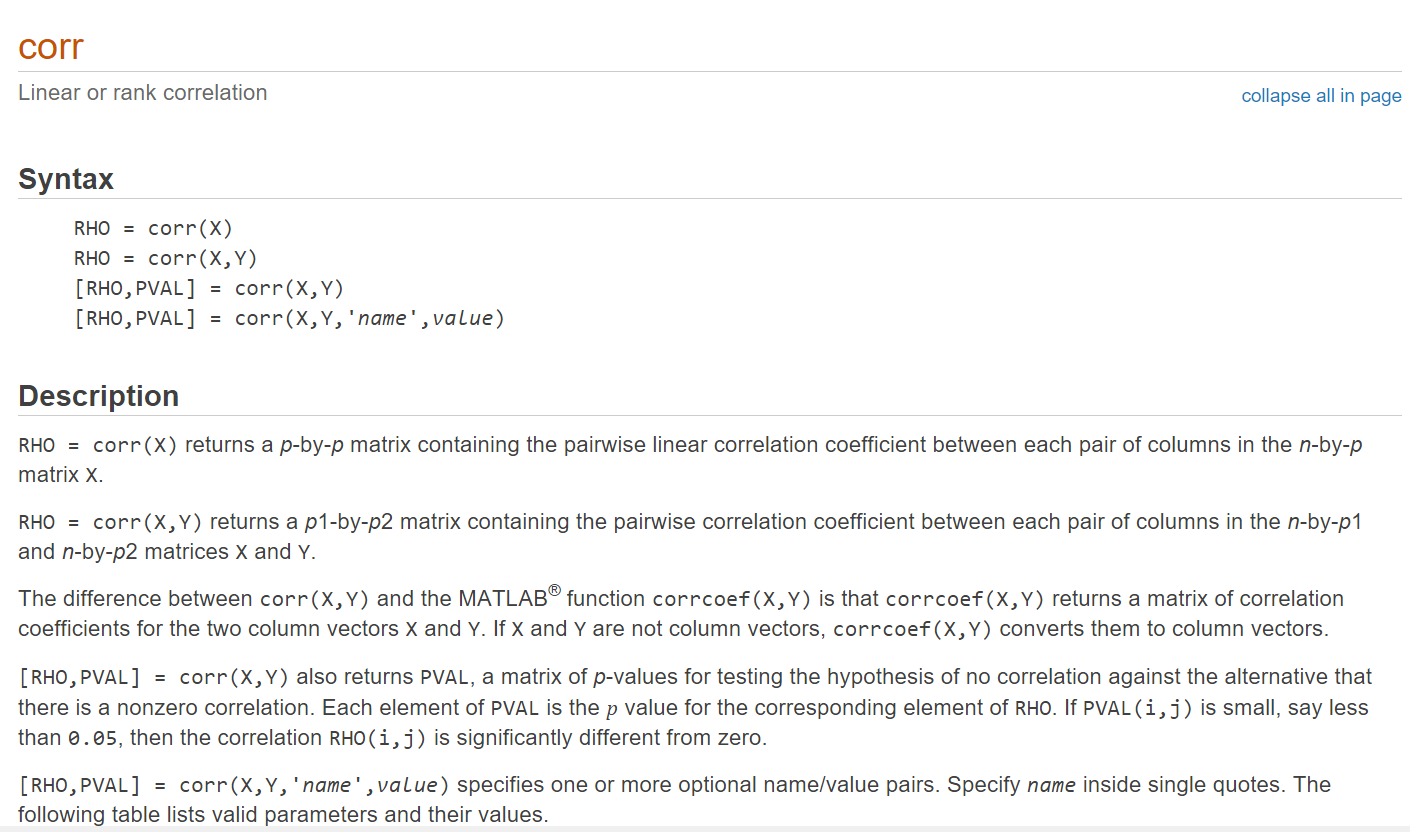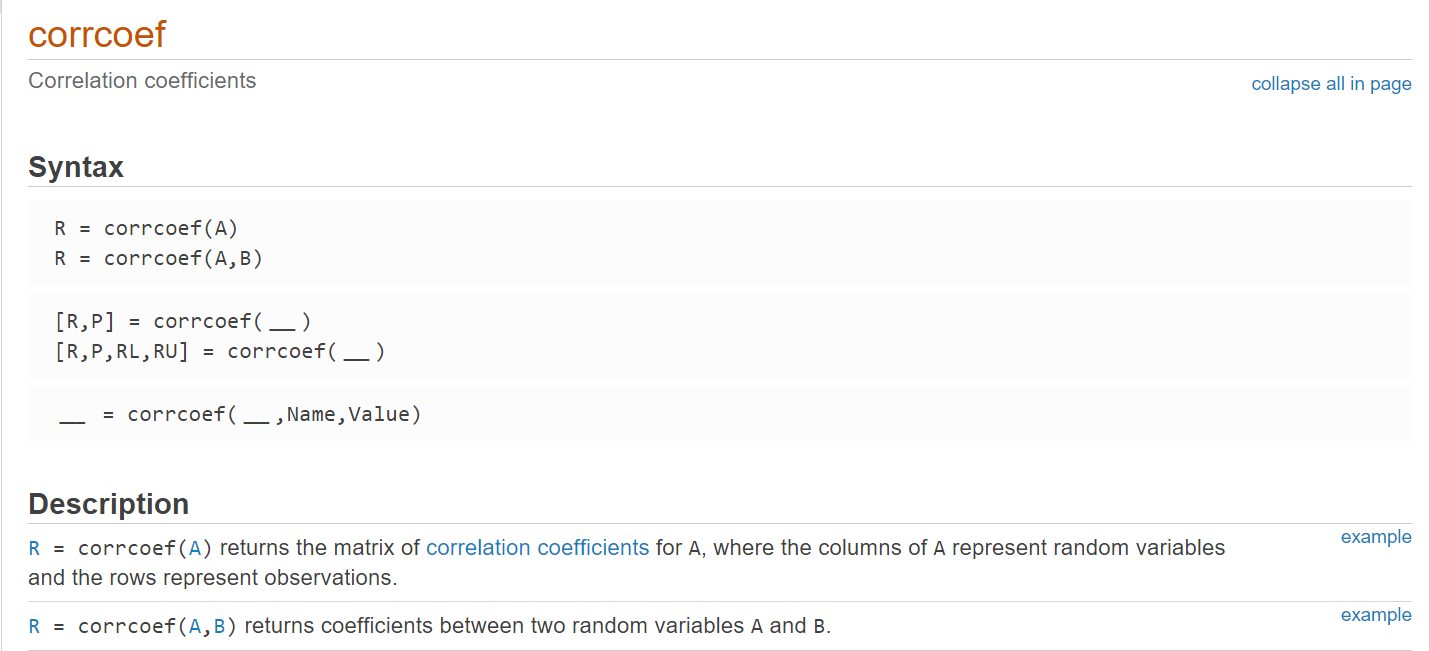• pearsonCorrelation(​Xp,Y_data,L):找到皮尔逊相关系数-matlab开发 matlab

1KB weixin_38547421 2021-05-29 20:03:53
• 皮尔逊相关系数计算（Matlab和Python实现） python matlab 开发语言

Matlab 注意，数组元素之间用分号隔开的 clear all; a=[ 0.6; 0.9;0.78; 0.28;0.44;0.40;0.69;0.94;0.45] b=[ 31.46;26.75;32.08 ;26.38;29.75;27.57;28.56 ;27.67 ;28.18 ] coeff = corr(a , b,'type','pearson'); ...

Matlab

注意，数组元素之间用分号隔开的

clear all;
a=[ 0.6; 0.9;0.78; 0.28;0.44;0.40;0.69;0.94;0.45]
b=[ 31.46;26.75;32.08 ;26.38;29.75;27.57;28.56 ;27.67 ;28.18 ]
coeff = corr(a , b,'type','pearson');Python

from  math  import  sqrt

def  multipl(a,b):
sumofab = 0.0
for  i  in  range ( len (a)):
temp = a[i] * b[i]
sumofab  =sumofab + temp
return  sumofab

def  corrcoef(x,y):
n = len (x)
#求和
sum1 = sum (x)
sum2 = sum (y)
#求乘积之和
sumofxy = multipl(x,y)
#求平方和
sumofx2  =  sum ([ pow (i, 2 )  for  i  in  x])
sumofy2  =  sum ([ pow (j, 2 )  for  j  in  y])
num = sumofxy - ( float (sum1) * float (sum2) / n)
#计算皮尔逊相关系数
den = sqrt((sumofx2 - float (sum1 ** 2 ) / n) * (sumofy2 - float (sum2 ** 2 ) / n))
return  num / den

#神经质维度
x  =  [ 0.6 , 0.9 , 0.78 , 0.28,0.44,0.40,0.69,0.94,0.45 ]
y  =  [ 31.46,26.75,32.08 ,26.38 ,29.75 ,27.57 ,28.56 ,27.67 ,28.18 ]

print (corrcoef(x,y))  #0.14418073993882827

from scipy.stats import pearsonr
pccs = pearsonr(x, y)
print(pccs)

(0.14418073993881686, 0.7113182029255729)

参考：

展开全文ningmengshuxiawo 2021-11-03 16:25:52
• 统计相关系数(1)——Pearson(皮尔逊)相关系数及MATLAB实现 算法 数据结构 C matlab python

统计相关系数(1)——Pearson(皮尔逊)相关系数MATLAB实现 标签： matlabfunction 2010-07-11 18:45 82823人阅读 评论(9) 收藏 举报  分类： MATLAB（6）  版权声明：本文为博主原创...

统计相关系数(1)——Pearson(皮尔逊)相关系数及MATLAB实现

82823人阅读  评论(9)  收藏  举报分类：

统计相关系数简介

由于使用的统计相关系数比较频繁，所以这里就利用几篇文章简单介绍一下这些系数。

相关系数：考察两个事物（在数据里我们称之为变量）之间的相关程度。

如果有两个变量：X、Y，最终计算出的相关系数的含义可以有如下理解：

(1)、当相关系数为0时，X和Y两变量无关系。

(2)、当X的值增大（减小），Y值增大（减小），两个变量为正相关，相关系数在0.00与1.00之间。

(3)、当X的值增大（减小），Y值减小（增大），两个变量为负相关，相关系数在-1.00与0.00之间。

相关系数的绝对值越大，相关性越强，相关系数越接近于1或-1，相关度越强，相关系数越接近于0，相关度越弱。

通常情况下通过以下取值范围判断变量的相关强度：
相关系数     0.8-1.0     极强相关
0.6-0.8     强相关
0.4-0.6     中等程度相关
0.2-0.4     弱相关
0.0-0.2     极弱相关或无相关

Pearson（皮尔逊）相关系数

1、简介

皮尔逊相关也称为积差相关（或积矩相关）是英国统计学家皮尔逊于20世纪提出的一种计算直线相关的方法。

假设有两个变量X、Y，那么两变量间的皮尔逊相关系数可通过以下公式计算：

公式一：公式二：公式三：公式四：以上列出的四个公式等价，其中E是数学期望，cov表示协方差，N表示变量取值的个数。

2、适用范围

当两个变量的标准差都不为零时，相关系数才有定义，皮尔逊相关系数适用于：

(1)、两个变量之间是线性关系，都是连续数据。

(2)、两个变量的总体是正态分布，或接近正态的单峰分布。

(3)、两个变量的观测值是成对的，每对观测值之间相互独立。

http://blog.csdn.net/wsywl/article/details/5727327

3、Matlab实现

皮尔逊相关系数的Matlab实现（依据公式四实现）：

[cpp]  view plain  copy
1. function coeff = myPearson(X , Y)
2. % 本函数实现了皮尔逊相关系数的计算操作
3. %
4. % 输入：
5. %   X：输入的数值序列
6. %   Y：输入的数值序列
7. %
8. % 输出：
9. %   coeff：两个输入数值序列X，Y的相关系数
10. %
11.
12.
13. if length(X) ~= length(Y)
14.     error('两个数值数列的维数不相等');
15.     return;
16. end
17.
18. fenzi = sum(X .* Y) - (sum(X) * sum(Y)) / length(X);
19. fenmu = sqrt((sum(X .^2) - sum(X)^2 / length(X)) * (sum(Y .^2) - sum(Y)^2 / length(X)));
20. coeff = fenzi / fenmu;
21.
22. end %函数myPearson结束

也可以使用Matlab中已有的函数计算皮尔逊相关系数：

[cpp]  view plain  copy
1. coeff = corr(X , Y);

4、参考内容

http://zh.wikipedia.org/zh-cn/%E7%9B%B8%E5%85%B3

http://blog.csdn.net/wsywl/article/details/5727327

相关书籍：考研必备参考：零基础学数据结构（第2版），机械工业出版社，2014，https://item.jd.com/10940947658.html，CC++函数与算法速查速解大辞典，中国铁道出版社，2015，https://item.jd.com/11770080.html，cc++数据结构与算法速学速用大辞典，中国铁道出版社，2016，https://item.jd.com/11983542.html，展开全文crcr 2017-02-28 14:13:35
• 由于使用的统计相关系数比较频繁，所以这里就利用几篇文章简单介绍一下这些系数相关系数：考察两个事物（在数据里我们称之为变量）之间的相关程度。 如果有两个变量：X、Y，最终计算出的相关系数的含义可以...

转自：http://blog.csdn.net/wsywl/article/details/5727327

由于使用的统计相关系数比较频繁，所以这里就利用几篇文章简单介绍一下这些系数。

相关系数：考察两个事物（在数据里我们称之为变量）之间的相关程度。

如果有两个变量：X、Y，最终计算出的相关系数的含义可以有如下理解：

(1)、当相关系数为0时，X和Y两变量无关系。

(2)、当X的值增大（减小），Y值增大（减小），两个变量为正相关，相关系数在0.00与1.00之间。

(3)、当X的值增大（减小），Y值减小（增大），两个变量为负相关，相关系数在-1.00与0.00之间。

相关系数的绝对值越大，相关性越强，相关系数越接近于1或-1，相关度越强，相关系数越接近于0，相关度越弱。

通常情况下通过以下取值范围判断变量的相关强度：
相关系数     0.8-1.0     极强相关
0.6-0.8     强相关
0.4-0.6     中等程度相关
0.2-0.4     弱相关
0.0-0.2     极弱相关或无相关

Pearson（皮尔逊）相关系数

1、简介

皮尔逊相关也称为积差相关（或积矩相关）是英国统计学家皮尔逊于20世纪提出的一种计算直线相关的方法。

假设有两个变量X、Y，那么两变量间的皮尔逊相关系数可通过以下公式计算：

公式一：公式二：公式三：公式四：以上列出的四个公式等价，其中E是数学期望，cov表示协方差，N表示变量取值的个数。

2、适用范围

当两个变量的标准差都不为零时，相关系数才有定义，皮尔逊相关系数适用于：

(1)、两个变量之间是线性关系，都是连续数据。

(2)、两个变量的总体是正态分布，或接近正态的单峰分布。

(3)、两个变量的观测值是成对的，每对观测值之间相互独立。

3、Matlab实现

皮尔逊相关系数的Matlab实现（依据公式四实现）：

[cpp]  view plain  copy

1. function coeff = myPearson(X , Y)
2. % 本函数实现了皮尔逊相关系数的计算操作
3. %
4. % 输入：
5. %   X：输入的数值序列
6. %   Y：输入的数值序列
7. %
8. % 输出：
9. %   coeff：两个输入数值序列X，Y的相关系数
10. %
11.
12.
13. if length(X) ~= length(Y)
14.     error('两个数值数列的维数不相等');
15.     return;
16. end
17.
18. fenzi = sum(X .* Y) - (sum(X) * sum(Y)) / length(X);
19. fenmu = sqrt((sum(X .^2) - sum(X)^2 / length(X)) * (sum(Y .^2) - sum(Y)^2 / length(X)));
20. coeff = fenzi / fenmu;
21.
22. end %函数myPearson结束

也可以使用Matlab中已有的函数计算皮尔逊相关系数：

[cpp]  view plain  copy

1. coeff = corr(X , Y);

4、参考内容

http://zh.wikipedia.org/zh-cn/%E7%9B%B8%E5%85%B3

转载于:https://www.cnblogs.com/baiting/p/5532202.html

展开全文weixin_34336292 2016-05-26 18:17:00
• 皮尔逊相关系数可以用来表述两个序列的相关性。 常常用来做数据分析、数据挖掘等工作。 如何简单快捷的实现皮尔逊相关，并得到结果？ 需要应用MATLAB中的corr(X, Y)或者 corrcoef(X,Y)函数。 其中corr(X, Y)既可以...

皮尔逊相关系数可以用来表述两个序列的相关性。

常常用来做数据分析、数据挖掘等工作。

如何简单快捷的实现皮尔逊相关，并得到结果？
需要应用MATLAB中的corr(X, Y)或者 corrcoef(X,Y)函数。

其中corr(X, Y)既可以计算矩阵相关也可以计算序列相关，而corrcoef(X,Y)中如果X, Y为矩阵，则会将其转换为序列再进行计算。

CORR伪代码

X,Y  # 为两个序列并且都是列向量.
pearson_corr = corr(X, Y)   #  求X, Y序列的皮尔逊相关系数.

该函数同样可以设置参数计算不同的相关系数。

'Pearson' (the default) computes Pearson's linear correlation coefficient
'Kendall' computes Kendall's tau
'Spearman' computes Spearman's rho

Example 1

A = randn(10,1);
B = randn(10,1);
R = corrcoef(A,B)
X = corr(A,B)

如果corr()输入为向量则其输出为两个向量相关性的标量系数。

R =
1.000000000000000   0.327514034455913
0.327514034455913   1.000000000000000
X =
0.327514034455913

即R（1，2）= R（2，1）= X

Example 2

rng('default')
x = randn(30,4);
y = randn(30,4);
y(:,4) = sum(x,2); % introduce correlation
[r,p] = corr(x,y)

其中 r 为两个矩阵中列向量间的相关系数，p 为非零相关的假设检验值（位置与 r 对应），如果值小于 0.05 则相关性显著。

r =

-0.1686   -0.0363    0.2278    0.6901
0.3022    0.0332   -0.0866    0.2617
-0.3632   -0.0987   -0.0200    0.3504
-0.1365   -0.1804    0.0853    0.4908

p =

0.3731    0.8489    0.2260    0.0000
0.1045    0.8619    0.6491    0.1624
0.0485    0.6039    0.9166    0.0577
0.4721    0.3400    0.6539    0.0059

CORRCOEF其中corrcoef( )返回的 P 值同样为假设检验值，在corr()中解释过不再解释，详情请见MATLAB帮助文档。

Example 1

x = randn(6,1);
y = randn(6,1);
A = [x y 2*y+3];
R = corrcoef(A)

上述例子中A为（6，3）矩阵，将其每列看作一组变量的序列，进行相关性计算，得到结果

R =
1.0000   -0.6237   -0.6237
-0.6237    1.0000    1.0000
-0.6237    1.0000    1.0000

对角线上由于是变量自相关所以都是1，元素(2,3)由于 y 与 2*y+3 是线性相关所以相关系数也是1。

Example 2

A = randn(10,1);
B = randn(10,1);
R = corrcoef(A,B)

上述A,B分别为长度为10的列向量，函数将两个列向量看作两个变量进行相关性分析。

R =
1.0000    0.4518
0.4518    1.0000

展开全文qq_37207042 2021-05-12 10:39:39
• weixin_42519826 2021-04-30 03:44:04
• 数学建模皮尔逊person相关系数和斯皮尔曼spearman等级相关系数Matlab实现代码 相关系数 斯皮尔曼相关系数 matlab 皮尔逊相关系数

qq_32589267 2019-07-27 16:34:53
• 皮尔逊相关系数 皮尔逊相关系数计算5星
3KB zhwb024 2011-11-12 20:35:14
• Pearson皮尔逊相关系数以及数据的描述性统计+Matlab代码 数据分析 matlab

weixin_51545953 2020-12-13 23:22:17
• 皮尔逊三型曲线matlab编程 皮尔逊三型

221KB cym30 2019-12-03 07:21:32
• matlab+python 热力图呈现：Pearson(皮尔逊)相关系数及MATLAB实现 matlab

weixin_45492560 2020-05-18 14:16:03
• Matlab计算皮尔逊相关系数corr、互相关系数xcorr 相关系数 皮尔逊相关系数 互相关系数

weixin_42617035 2019-11-12 14:28:40
• 1KB sdshiwangtong 2018-06-25 10:27:38
• lm_is_dc 2020-03-02 17:21:56
• weixin_39736913 2020-12-08 14:51:28
• gengjuan520 2021-04-05 16:47:45
• 数学建模 --- 皮尔逊相关系数 matlab 数学建模

qq_43779658 2020-08-03 01:41:00
• 数学建模——相关系数（1）——皮尔逊相关系数（Person） 数学建模

NoBuggie 2019-10-21 16:01:03
• 数学建模方法——皮尔逊相关系数及其显著性检验 （Pearson correlation coefficient） 正态分布检验 jb检验

limiyudianzi 2019-12-07 16:47:36
• yanyanwenmeng 2020-12-11 16:54:10
• a_pickles 2020-07-14 20:56:12
• wenyusuran 2014-11-04 10:07:36
• 如何通俗易懂地理解皮尔逊相关系数？ 皮尔逊相关系数 协方差 数理统计与数据分析 相关系数

huangfei711 2017-11-06 11:45:57
• 【数学建模】相关性分析 - 皮尔逊相关系数 & 斯皮尔曼相关系数 数学建模

Aloneingchild 2020-09-18 23:51:36...

皮尔逊相关系数matlabmatlab 订阅